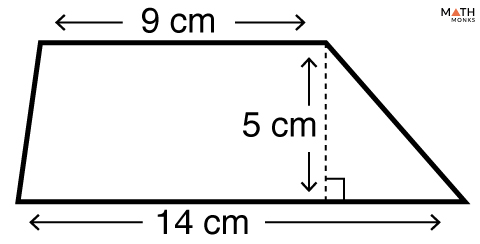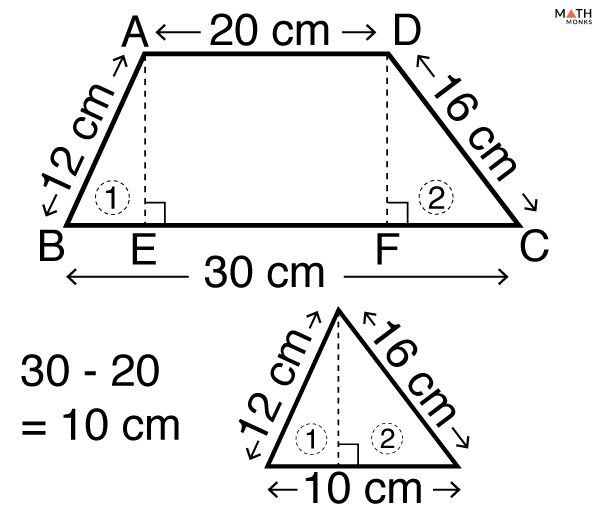# Area of a Trapezoid

The area of a trapezoid is the total space enclosed by it. Since a trapezoid is a two-dimensional shape, its area also lies in a two-dimensional plane. The unit of area is in square units such as m2, cm2, in2 , or ft2.

## Formula

### Using Height and Base Lengths

The basic formula to calculate the area of a trapezoid is given below:

Let us solve an example to understand the concept better.Find the area of a trapezoid whose base lengths are 14 cm and 9 cm, and height is 5 cm.

Solution:

As we know,
Area (A) = ½ (a + b) × h, here a = 14 cm, b = 9 cm, and h = 5 cm
∴A = ½ (14 + 9) × 5
= 57.5 cm2

### Without Height

We can calculate the area of a trapezoid when all 4 sides are known. There is no definite formula as such for such a circumstance. We try to calculate the area of the triangle as a portion formed in a trapezoid and then calculate the whole area of the trapezoid.

Let us solve an example to understand the concept better.

Problem: Finding the area of a trapezoid when the SIDES are known.Find the area of a non-isosceles trapezoid whose bases are 20 and 30, and the legs 12 and 16.Solution:

Let us label the trapezoid ABCD and mark the triangles Δ1 and Δ2, formed by 2 perpendiculars drawn in.
As we know,
Long base is 30 cm,
∴BE + FC = long base – short base
= 30 – 20
= 10 cm
Δ1 and Δ2 together forms a scalene triangle (3 unequal side lengths).
Here, we will use the Heron’s Formula to find the area of Δ1 and Δ2 together so as to find the perpendicular height of the trapezoid.
Area of Δ1 and Δ2  ${ =\sqrt{s\left( s-a\right) \left( s-b\right) \left( s-c\right) } }$ , here s = ½(a + b + c)
∴s = ½(a + b + c), here a = 10 cm, b = 12 cm, c = 16 cm
s = ½(10 + 12 + 16)
= 19 cm
Now, Area of Δ1 and Δ2 ${ =\sqrt{s\left( s-a\right) \left( s-b\right) \left( s-c\right) } }$ , here s = 19 cm
= √[19(19 – 10)(19 – 12)( 19 – 16)]
= 59.92 cm2
Height (h) of a scalene triangle = 2A/b, here A = area, b = base
∴h = 2A/b, here A = 59.92 cm2, b = 10 cm
∴h = 2 × 59.92/10
= 11.984 cm
Now, Area of trapezoid ABCD = ½ (a + b) × h, here a = 30 cm, b = 20 cm, h = 11.984 cm
∴A = ½ (30 + 20) × 11.984
= 299.6 cm2